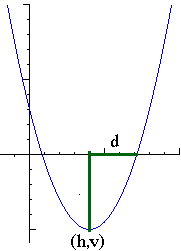Mind your p's and q's!by Henri Picciotto

Links to many lessons and activities, to be used in Algebra 1, Algebra 2, and with math teachers, including three paths to the quadratic formula.

Look at everything, and decide what goes where for you, as the headings are merely guidelines.

Mostly Algebra 1

A manipulatives-based complement to this work (or to any work on quadratics) can be found in my book Algebra Lab Gear: Algebra 1, available from Didax. The manipulatives make factoring and completing the square accessible to the vast majority of Algebra 2 students, while giving them a geometric perspective which is unfortunately missing from many curricula. That approach is all the more important if you teach this material to students in grades 7-9.
- Sample activity from the end of the book, which makes a connection between the Lab Gear and graphical representations of certain quadratics (PDF).
- Applet to preview and discuss those connections.
- Applet that presents a geometric view of completing the square. (Read this first.)
- Related GeoGebra files: trinomial | trinomial square
An apparently unrelated unit: Constant Sums, Constant Products
Images that reveal many connections.
Related GeoGebra file
An application of quadratic functions, from Algebra: Themes, Tools, Concepts:
Maximizing Area | Condensed version.

Mostly Algebra 2

The lessons below (in PDF format) involve the sparing use of graphing technology. Unlike some other tech-based approaches ("graph this, graph that, what do you notice?") this is a rather theoretical unit, which helps develop students' symbol sense and goes for a lot more depth of understanding than is customarily expected at this level.
Factored Form of Quadratic Functions | Applet | Geogebra file
From Factored to Standard Form
GeoGebra file to explore the effect of varying a, b, or c.
Moving Parabolas Around
Quadratic Forms (a condensed version of the above three lessons, probably better suited for teachers)
Make These Parabolas: TI-83/84, TI-89
Related GeoGebra file: Translating Parabolas
A derivation of the quadratic formula using graphs:
A Graphical Approach to the Quadratic Formula
Related GeoGebra file
It is especially useful if you eschew the manipulatives / geometric approach to completing the square, or emphasize transformations of graphs, but you will find it interesting no matter what.

Calculus

A student-created visual proof: Integrating y=x2, by Jacob Regenstein.

Mostly for Teachers

Late in the Math 1 / Algebra 1 course at The Urban School, where I used to work, I asked students to create a short report explaining the connections illustrated here, using their own example. One year, while grading this assignment, it hit me that there must be a new proof of the quadratic formula based on this, and sure enough, there is. You can read it in my article in the February 2008 issue of The Mathematics Teacher, under the title "A New Path to the Quadratic Formula". The proof involves neither parabolas nor completing the square.
Worksheet (for teachers and perhaps some precalculus students)
(background: Constant Sums, Constant Products)
Related Cabri and GeoGebra files
See an online or Keynote presentation of the proof. Either way, grab a pencil to follow the argument, and click your mouse to advance through the slides.
Parabolas are a major topic in secondary school, and yet in the US, students and teachers do not often think about them as geometric objects. This may be a consequence of the strict division between algebra and geometry courses. As a result, many basic properties of parabolas are not well understood. These pages present some key concepts in this domain:
Geometry of the Parabola: 2D | Parabola Similarity | 3DRelated pages on this site:

Subscribe to my newsletter